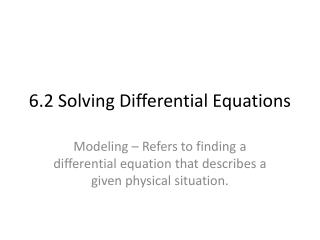Download Presentation6.2 Solving Differential Equations6.2 Solving Differential Equations - PowerPoint PPT Presentation

Download Presentation6.2 Solving Differential Equations
An Image/Link below is provided (as is) to download presentation

Download Policy: Content on the Website is provided to you AS IS for your information and personal use and may not be sold / licensed / shared on other websites without getting consent from its author. While downloading, if for some reason you are not able to download a presentation, the publisher may have deleted the file from their server.

- - - - - - - - - - - - - - - - - - - - - - - - - - - E N D - - - - - - - - - - - - - - - - - - - - - - - - - - -
Presentation Transcript

1. 6.2 Solving Differential Equations Modeling – Refers to finding a differential equation that describes a given physical situation.

2. Not too difficult, something I think is helpful is when you see y’ in an equation change it to dy/dx. (Leibniz notation) • Process involves getting all x’s on one side, and all y’s on the other. We will treat dy/dx as a fraction and we can move the dy and dx with algebra.

3. Example

4. In a lot of applications, the rate of change of a variable y is proportional to the value of y. If y is a function of time t, the proportion can be written as shown. Rate of change of y Proportional to y is Constant of proportionality

5. Leibniz Notation Separation of variables Integrate both sides Natural log (on left side) Solve for y

6. C is the initial value of y, and k is the proportionality constant. Exponential growth occurs when k>0 and exponential decay occurs when k<0. • All solutions for y’=ky are of the form

7. The rate of change of y is proportional to y. When t = 0, y = 2. When t = 2, y = 4. What is the value of y when t = 3? • When t = 0 and y = 2 (initial condition because time is 0) When t=2 and y=4

8. Suppose that 10 grams of the plutonium isotope Pu-239 was released in the Chernobyl nuclear accident. How long will it take 10 grams to decay to 1 gram? (remember this is decaying so k will be < 0) • Let y be # of grams. Pu-239’s half life is 24,100 years Initial conditions. t = 0, y = 10 Initial Conditions to find C. Half life conditions

9. Half Life Model Find time it takes to decay to 1 gram, then y=1 80,059 years

10. Suppose an experimental population of fruit flies increases according to the law of exponential growth. There were 100 flies after the second day of the experiment and 300 flies after the fourth day. Approximately how many flies were in the original population? • The first problem was not too difficult because the initial conditions were given, here the initial conditions are not given. • Let y=Cekt be the number of flies at time t, where t is measured in days. Because y=200 when t=2 and y=300 when t=4, you can write 100=Ce2k and 300=Ce4k. Take and solve for C. Now substitute the new C into the second equation.

11. Substituting C into second equation and solving for k. Now solve for C by reapplying the condition that y=100 when t=2. Then take that C and plug into the formula y=Cekt and plug in the initial time t=0 to find out the initial population of fruit flies.

12. Newton’s Law of Cooling states that the rate of change in the temperature of an object is proportional to the difference between the object’s temperature and the temperature of the surrounding medium (air most of the time). Let y represent the temperature in Fahrenheit of an object in a room whose temperature is kept at a constant 60°. If the object cools from 100° to 90° in 10 minutes, how much longer will it take for its temperature to decrease to 80°? Integrate both sides

13. Use initial conditions of t=0 and y=100 to find C. Now when y=90 and t=10. (model)